# C104 – API Volumetric Flow Rate of Natural Gas at Standard Conditions (Factors Approach Method) 1992

## Description

This calculates the volumetric flow rate of natural gas at standard conditions using the ‘Factors Approach’ method outlined in the MPMS Chapter 14.3.3 Appendix 3-B.

FLOCALC Calculation reference F034

Kelton calculation reference C024

Kelton calculation reference C104

Manual of Petroleum Measurement Standards Chapter 14 – Natural Gas Fluids Measurement, Section 3 – Concentric, Square-Edged Orifice Meters, Part 3 – Natural Gas Applications, Appendix 3-B – Factors Approach (Third Edition 1992; Reprinted 2009)

## Calculations

Calculation as documented in standard

### Volumetric Flow Rate

The volumetric flow rate is calculated from: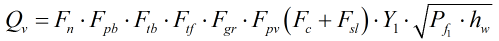Where Fc = Orifice calculation factor Fgr = Real gas relative density factor Fn = Numeric conversion factor Fpb = Base pressure factor Fpv = Supercompressibility factor Fsl = Orifice slope factor Ftb = Base temperature factor Ftf = Flowing temperature factor Y1 = Expansion factor Pf1 = Upstream pressure in psi hw = Orifice differential pressure in inH2O (60°F) C’ = Composite flow factor

### Orifice Calculation Factor

The numeric conversion factor, Fc, is calculated from: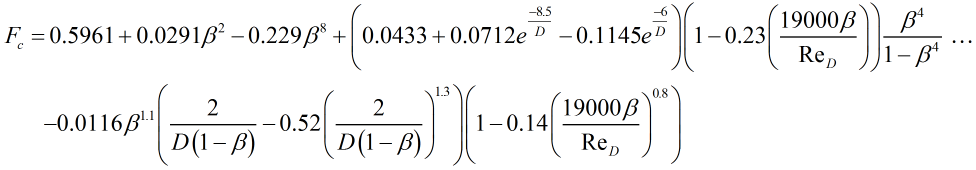Where D = Pipe diameter at flowing temperature in inches β = Orifice diameter ratio ReD = Reynolds number of pipe flow

### Real Gas Relative Density Factor

The real gas relative density factor, Fgr, is calculated from: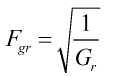Where Gr = Real gas relative density

### Numeric Conversion Factor

The numeric conversion factor, Fn, is calculated from: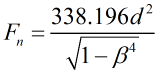Where d = Orifice bore diameter at flowing temperature in inches β = Orifice diameter ratio

### Base Pressure Factor

The base pressure factor, Fpb, is calculated from: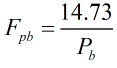Where Pb = Base pressure in psi

### Supercompressibility Factor

The supercompressibility factor, Fpv, is calculated from: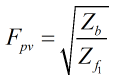Where Zb = Base supercompressibility factor Zf1 = Line supercompressibility factor

### Slope Factor

The slope factor, Fsl, is calculated from: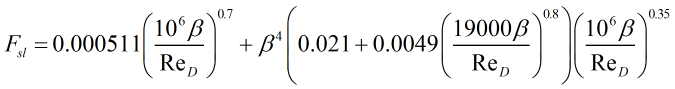Where β = Orifice diameter ratio ReD = Reynolds number of pipe flow

### Base Temperature Factor

The base temperature factor, Ftb, is calculated from: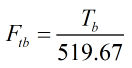Where Tb = Base temperature in °R

### Flowing Temperature Factor

The flowing temperature factor, Ftf, is calculated from: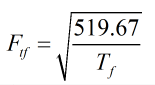Where Tf = Flowing temperature in °R

Back